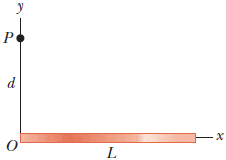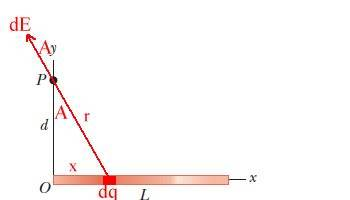# Electric Field of a uniformly charged rod

## Homework Statement

A uniformly charged rod of length L and total charge Q lies along the x axis as shown.Find the components of the electric field at the point P on the y-axis a distance d from the origin.
There's also a part b but I should be able to figure it out easily enough.

## Homework Equations

$\stackrel{\Downarrow}{E}=k_{e}\int\frac{dq}{r^{2}}\widehat{r}$

## The Attempt at a Solution

My instructor has not worked any exampled like this yet, and I'm really not sure how to begin.

Delphi51
Homework Helper
Mark off a small piece of the rod and label it dx, and its charge dq. You can express dq in terms of dx, the length L and the total charge on the rod.

Write the formula for the dE caused by dq at point P. Integrate it over the length of the rod - the integral will look very much like the equation your posted.

...formula for the dE caused by dq at point P...

Would I use

$F_{e}=k_{e}\frac{q_{1}q_{2}}{r^{2}}$

and

$E=\frac{F_{e}}{q_{0}}$

Thats the only equation we have that relates q and distance r, but I'm not sure what to actually plug in.

EDIT: I should plug in dq=(lambda)dL, where lambda is q/L?

Delphi51
Homework Helper
Yes, that all makes sense.
Put the two formulas together, eliminating Fe.

Your q0 will cancel q2 so your formula is E = k*q/r². This is a more fundamental formula, which surely you have on your list, for the electric field caused by a charge q around itself. Replace q with dq and E with dE in this situation. Integrate dE to get E.

Alright, so I have

$k\int\frac{dq}{L^{2}}$

My professor only worked one example, and it was used because it was symmetrical and most charges cancelled out.
I'm looking over the video he did, and he added a cos(theta) in the integral (his reasoning being "this needs to go here"). I'm assuming I will need something like that to show the change in the angle, but not sure where.

Delphi51
Homework Helper
Good catch! I forgot about the angle. Both the "r" in E = k*q/r² and the direction of dE depend on the angle A. (Sorry, can't make a theta in my paint program.)You can avoid the first; just express your r in terms of d and x.
Unfortunately there is no symmetry in this problem to cancel out the horizontal part of the field. You can't add contributions to the E field in different directions, so you will have to do the horizontal and vertical components of E separately, with two integrals. One will have a cos A and the other a sin A factor. This brings up another difficulty. The x, r and A all vary! Too many variables. You'll have to pick one for dx, dr or dA and express the other two in terms of the one you choose. I kind of like x for the variable, but it does make cos A and sin A a little awkward. Hope you have an integral table handy. Or www.integrals.com.

I can get r in terms of x and d:

$r=x^{2}+d^{2}$

I guess I put that in the integral:

$k\int\frac{dq}{x^{2}+d^{2}}$

I know thats not right, I really have no idea, I'm giving up on this problem.

We have 12, all were super easy (variations of E=F/q) except this one.

I don't even partly understand what the heck I'm doing, and all my instructor will do is point me to his 5 minute video of him working out (and skipping most of the steps) a simple problem.

Thanks for your help, and sorry about the rant. I've literally been looking at this problem for over 6 hours!

Last edited:
Delphi51
Homework Helper
Sorry, I had things to do.
We're getting pretty close; might be worth finishing if the integral is in the table.
I have dE = kdq/r², dq = q/L*dx, cos(A) = d/r
For the vertical component of E, use dE*cos(A) = kqd/L/r³*dx
The vertical component of E is kqd/L Integral of dx divided by (x² + d²)³/²
Is that baby in your integral table?

Last edited: